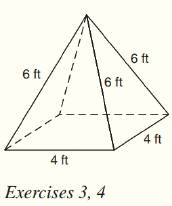Chapter 9.CT, Problem 3CTElementary Geometry For College St...

7th Edition
Alexander + 2 others
ISBN: 9781337614085

Solutions

Chapter
SectionElementary Geometry For College St...

7th Edition
Alexander + 2 others
ISBN: 9781337614085
Textbook Problem

For the regular square pyramid shown, find the total number of a) vertices. b) lateral faces.To determine

a)

To find:

The total number of vertices in the regular square pyramid.

Explanation

Given:

The regular square pyramid is shown below

Calculation:

From the figure we see that the base is a square.

There are four vertices in a square...

To determine

b)

To find:

The total number of lateral faces in the regular square pyramid.

Still sussing out bartleby?

Check out a sample textbook solution.

See a sample solution

The Solution to Your Study Problems

Bartleby provides explanations to thousands of textbook problems written by our experts, many with advanced degrees!

Get Started

Find more solutions based on key concepts